# Beyond 0-1 choices¶

• Graphical game

• $x_i$ in [0,1]

• Start with Yann Bramoulle and Rachel Kranton: information acquisition

# Bramoulle-Kranton Setting¶

• payoff: $f(x_i + \sum_{j \in N_i(g)} x_j) - c x_i$

• concave function
• c: each person pays a per-unit cost of their effort
• Take derivative of it, let $x^* > 0$ solve $f'(x^*) - c =0$

• In all pure strategy Nash equilibria:

• $x_i + \sum_{j \in N_i(g)} x_j \ge x^*$ for all i, and if >, then $x_i = 0$
• Look at two types of pure equilibria

• distributed: $x^* > x_i >0$ for some i's
• specialized: for each i either $x_i=0$ or $x_i=x^*$

# Various Equilibria¶

• Case $x^* = 1$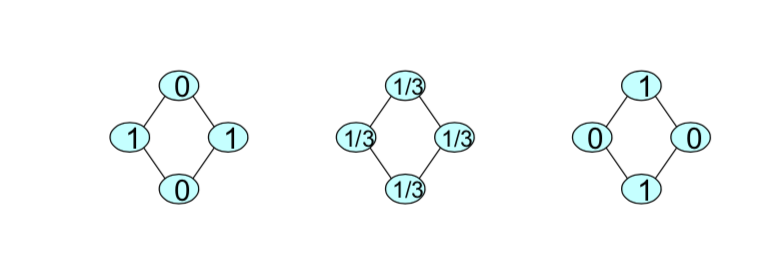Left pic:

Here's a situation where we've got a specialized equilibrium. These people provide the full action, their neighbors free ride. Everybody gets exactly x star in their neigbhorhood. Nobody wants to increase their action. in fact, some people. These individuals get more than x star. They get two times. So they're happy. They're getting double the provision of public goods. They certainly don't want to contribute anything. And these people are getting exactly one, they're providing it because their neighbors are not providing anything, there's another equilibrium.

Middle pic:

Here's another equilibrium. Everybody provides 1/3. And that balances because we're in a situation where each person is seeing exactly x star in their neighborhood, so nobody wants to increase or decrease. Right? So this is a distributed one, where we've got an exact distribution.

Right pic: It's similar to the Left pic, but just with roles reversed.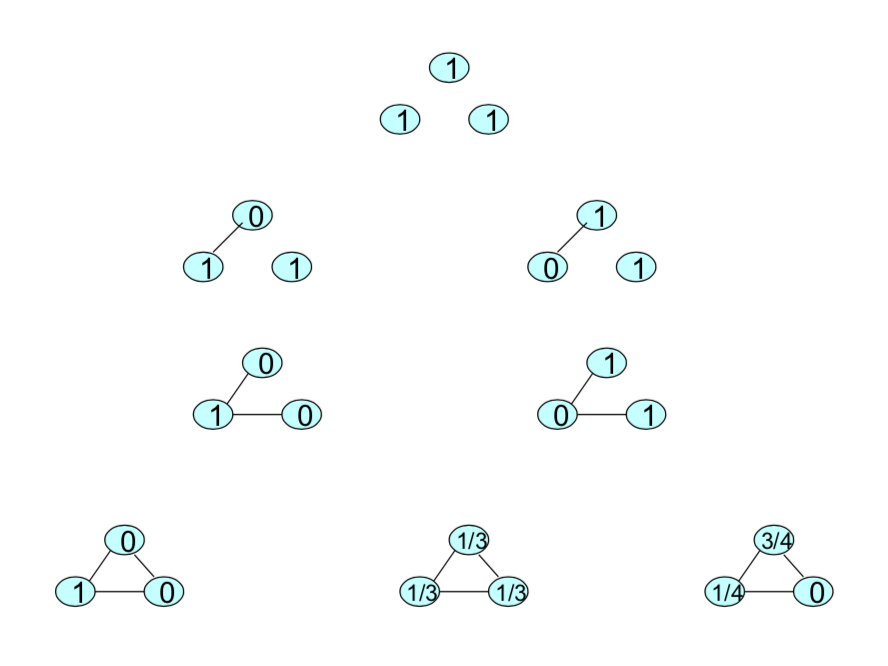# Specialization Equilibria¶

• Maximal independent set - set S of nodes such that

• no two nodes in S are linked
• every node in N is either in S or linked to a node in S
• Proposition(B&K): The set of specializated Nash equilibria are profiles such that a maximal independent set = the specialists($x_i = x^*$)

# Stability Notion: pure strategy equilibrium¶

• perturb x to $x^0 = (x_1 + \epsilon_1, ... x_n + \epsilon_n)$, being sure that all entries are feasible. $\epsilon$ can be positive or negative.

• Let $x^1$ be the best response to $x^0$, $x^t$ to $x^{t-1}$

• If for all small enough $\epsilon_i's$ converge back to x, then "stable"

# Stable equilibria¶

• Dyad: nothing is stable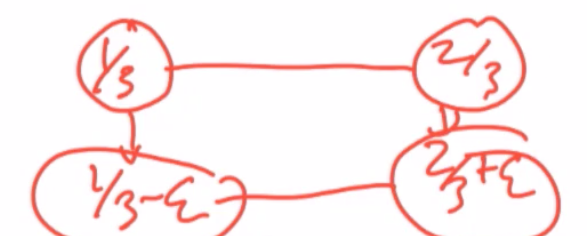so we've got two individuals, these two individuals, and let's suppose that, you know, person 1 is doing something say like a 1 3rd and person 2 doing 2 3rds. that's not going to be stable in the sense that if we perturb this a little bit, so suppose we move this down to 1 3rd, minus epsilon and this up to 2 3rds plus epsilon, well that in fact is also an equilibrium. So if we did that perturbation it wouldn't go back to the original one, it would actually stay at this one, okay? So in a, in a dyad, no matter what numbers you put in there, you know, we could put in zero and one, and, and have this go to 0 plus epsilon, 1 minus epsilon so no matter what numbers you put in, as you perturb this, it would, it would stay there.

• let $x_1 \le x_2$

• $x_1 + \epsilon, x_2 - \epsilon$ stays there

So as long as x one is less than or equal to x two. Add a little bit to x one. And subtract a little bit from x two. You would stay there. So you're in a situation where you end up not having, any movement in terms of, of the perturbation. nothing's stable. Okay, But when we look more generally. the equilibria are, the only stable equilibria aren't necessarily going to be specialist equilibria. And they're going to be such that every non-specialist has exactly, at least two specialists in his or her neighborhood. So, for instance, if we look at three nodes, and again, our x star is one. This thing is stable.

# Stable equilibria, BK¶

• Only stable equilibria are specialist equilibria such that every non-specialist has two specialist in his or her neighborhood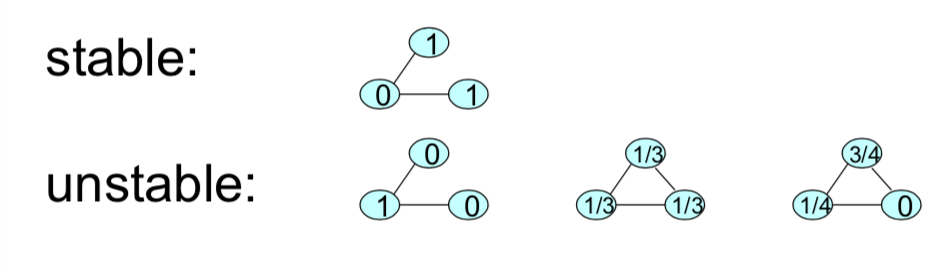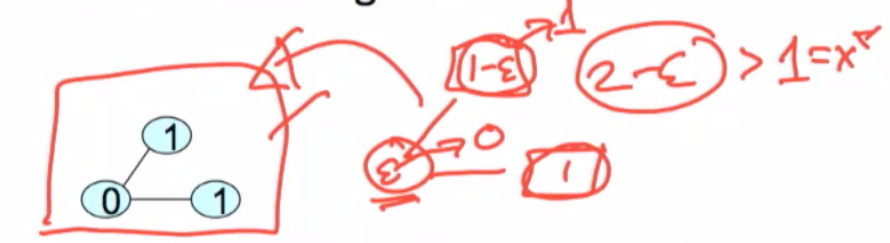let's just go through and try to understand why is this stable? What's the logic, here? So, let's suppose that we perturb this a little bit. So for instance we take a little bit of $\epsilon$ away, so we take this person down to $1 - \epsilon$. And we bump this person up to $\epsilon$. And let's just keep this person constant for now. if we do that, and now we look at the best responses to this. Well, this person has already a neighbor providing at least one. They're still at $2 - \epsilon$ in their neighborhood. They're way bigger than the $x^*$ of one. They're getting too much action. They can get rid of their $\epsilon$ and go back to zero. So, they're going to tend to go right back to zero. Once they go back to zero, this person's going to go back to one, and we end up going back to the situation that we have.

So here the fact that I'm already getting too much means even if you perturb that I'm going to want to go right back to zero. So the people that are in the neighborhood of these specialists are going to stay there if they have at least two specialists in their neighborhood they're going to go back to zeroes. And that means that specialists are going to be forced back to one because all their neighbors are providing zeroes.

# Sketch of Proof¶

• Stability of such equilibria: for small perturbations, BR of non-specialists is 0, converge right back

• For any other equilibrium, if there is an agent providing is non-specialists, then peturb the agent up, neighbors go down...

• If all specialists or not - then some non-specialist just has one neighbor as specialist - raise that nonspecialist, lower the specialist...

# Stability and Pairwise Stability¶

• Suppose links are costly

• specialists drop links to non

• non-specialized equilibria are only "stable" ones...

# Heterogeneity¶

• Introduction of heterogeneous costs and benefits, and some less than perfect spillovers

• Would change the nature of equilibria